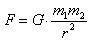# What is the universal constant of gravity

## Gravitational constant

Gravitational constant, 1) Newtonian constant of gravity G, Proportionality factor im Newton's law of gravitationthat the force F. between two masses m1 and m2 at a distance r each other describes with G = (6,672 59 ± 0,000 85) · 10-11N · m2 / kg2. In order to determine it, a laboratory experiment is necessary, in which the masses and spacing of the specimens are precisely known. The first experiment of this kind was carried out by Cavendish in 1798 with a rotary balance.

In some cosmological hypotheses, G not viewed as a fundamental constant, but as changing over time.

2) Einstein's constant of gravity κ, in the General Relativity used constant κ = 8π G / c4 = 2,076 12 · 10-43 s2 / (m kg).

3) Gaussian constant of gravity k, in the third Kepler's law (the square of the period of revolution of a body around a central star of mass m2 is proportional to the cube of its mean distance um m2) Part of the constant 4π2 / k2m2. The name used in astronomy comes from the fact that in the relationshipthe numerical values ​​of, ω and k are the same if the mass of the sun, the astronomical unit AE and the day are used as a unit. (astronomical constants)